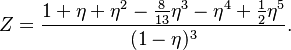2022: SklogWiki celebrates 15 years on-line

Liu hard sphere equation of state

(diff) ← Older revision | Latest revision (diff) | Newer revision → (diff)$Z = \frac{ 1 + \eta + \eta^2 - \frac{8}{13}\eta^3 - \eta^4 + \frac{1}{2}\eta^5 }{(1-\eta)^3 }.$